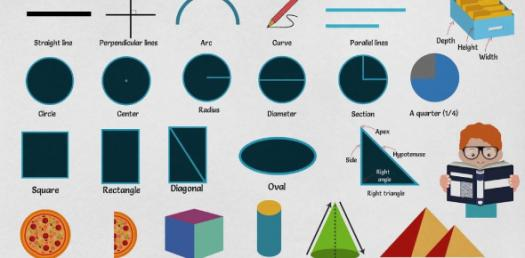# Math Vocabulary 5th Grade Unit 3

6 Questions | Total Attempts: 103SettingsVolcabulary 5th grade quiz - Kaitlyn

• 1.
Match correct meaning of CENSUS
• A.

Measures between 0 degrees and 90 degrees

• B.

Measures between 90 degrees and 180 degrees

• C.

Comes from the Latin word meaning "to tax" or "to appraise"

• D.

Measures between 180 degrees and 360 degrees

• 2.
Match correct meaning of ACUTE ANGLE
• A.

Measures between 90 degrees and 180 degrees

• B.

Measures between 180 degrees and 360 degrees

• C.

Measures 90 degrees

• D.

Measures between 0 degrees and 90 degrees

• E.

Measures between 360 degrees and 720 degrees

• 3.
Match correct meaning of OBTUSE ANGLE
• A.

Measures between 0 degrees and 90 degrees

• B.

Measures between 90 degrees and 180 degrees

• C.

Measures between 180 degrees and 360 degrees

• D.

Measures between 360 degrees and 720 degrees

• E.

Measures 90 degrees

• 4.
Match correct meaning of RIGHT ANGLE
• A.

Measures 90 degrees

• B.

Measures between 0 degrees and 90 degrees

• C.

Measures between 90 degrees and 180 degrees

• D.

Measures between 180 degrees and 360 degrees

• E.

Measures between 360 degrees and 720 degrees

• 5.
Match correct meaning of REFLEX ANGLE
• A.

Measures 90 degrees

• B.

Measures between 0 degrees and 90 degrees

• C.

Measures between 90 degrees and 180 degrees

• D.

Measures between 180 degrees and 360 degrees

• E.

Measures between 360 degrees and 720 degrees

• 6.
Match correct meaning of GEOMETRY TEMPLATE
• A.

Comes from the Latin word meaning "to tax" or "to appraise"

• B.

Has 2 rulers - inch and centimeter scale

• C.

A tool used to measure angles

• D.

A flat, 2-dimensional figure made up of line segments

• E.

Has 17 different figures on it

Related TopicsBack to top